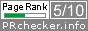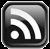# LEARN MS-ACCESS TIPS AND TRICKS

Learn Microsoft Access Advanced Programming Techniques, Tips and Tricks.

### Introduction.

Microsoft Access database can be a maximum of 2GB in size. We can back up old Records (like previous year transactions) into a Backup database safely for later use if the need arises. After the safe backup of these transactions, they can be deleted from the active database and Run Compact & Repair Utility to optimize the database performance in day-to-day activities.

There are times that we need the old data for analysis purposes, like next year's budgeting, sales target setting, and so on. At this point we will need old records to combine with the existing data of the active master-table, in the current database, to do various analysis runs.

### The Union Query Solution.

We can merge records of both the active table and backup table (with the same name) in a Union Query.

A simple example is given below:

```SELECT Export.*
FROM Export
UNION ALL SELECT Export.*
FROM Export IN 'G:\NEW FOLDER\DB1.MDB';
```

From the above example, you can see that the name of the table Export in an active database and backup database(DB1.MDB) are the same. No need to link the table to the active database to access the data from the backup database.

Share:

### Introduction.

Microsoft Access doesn't have this function built-in. I have made an attempt to write the code for this function and you may use it at your own risk. Before going to the code take a look at the usage examples of this function in Excel.

### ROUNDUP() function in Microsoft Excel.

#### The Rules.

Rounds a number up, away from 0 (zero).

Syntax: ROUNDUP(number,num_digits) Number is any real number that you want to be rounded up.

Num_digits is the number of digits to which you want to round number.

Remarks ROUNDUP behaves like ROUND, except that it always rounds a number up.

If num_digits is greater than 0 (zero), then the number is rounded up to the specified number of decimal places.

If num_digits is 0, then the number is rounded up to the nearest integer.

If num_digits is less than 0, then the number is rounded up to the left of the decimal point.

#### Examples:

=ROUNDUP(3.2,0) Rounds 3.2 up to zero decimal places (4)

=ROUNDUP(76.9,0) Rounds 76.9 up to zero decimal places (77)

=ROUNDUP(3.14159, 3) Rounds 3.14159 up to three decimal places (3.142)

=ROUNDUP(-3.14159, 1) Rounds -3.14159 up to one decimal place (-3.2)

=ROUNDUP(31415.92654, -2) Rounds 31415.92654 up to 2 decimal places to the left of the decimal (31500)

Courtesy: Microsoft Excel Help Documents.

Copy and Paste the following VBA Code into a Standard Module of your Database and try it out. The examples given above have been successfully passed on my testing of the code.

### The ROUNDUP() Function.

```Public Function ROUNDUP(ByVal dblNum As Double, ByVal intprecision As Integer) As Double
'-------------------------------------------------
'ROUNDUP() Function of Excel Redefined in MS-Access
'Author: apr pillai
'Date  : June 2016
'Rights: All Rights Reserved by www.msaccesstips.com
'-------------------------------------------------
On Error GoTo ROUNDUP_Err
Dim sign As Integer
sign = Sgn(dblNum)
dblNum = Abs(dblNum)
dblNum = dblNum * 10 ^ intprecision
ROUNDUP = (Int(dblNum + (1 - IIf((dblNum - Int(dblNum)) <> 0, (dblNum - Int(dblNum)), 1))) / 10 ^ intprecision) * sign

ROUNDUP_Exit:
Exit Function

ROUNDUP_Err:
MsgBox Err & ": " & Err.Description, , "ROUNDUP()"
Resume ROUNDUP_Exit
End Function```

suggestions for improvement of the above VBA Code are welcome.

Share:

## TranslateSubscribe in a readerYour email address:

Delivered by FeedBurner